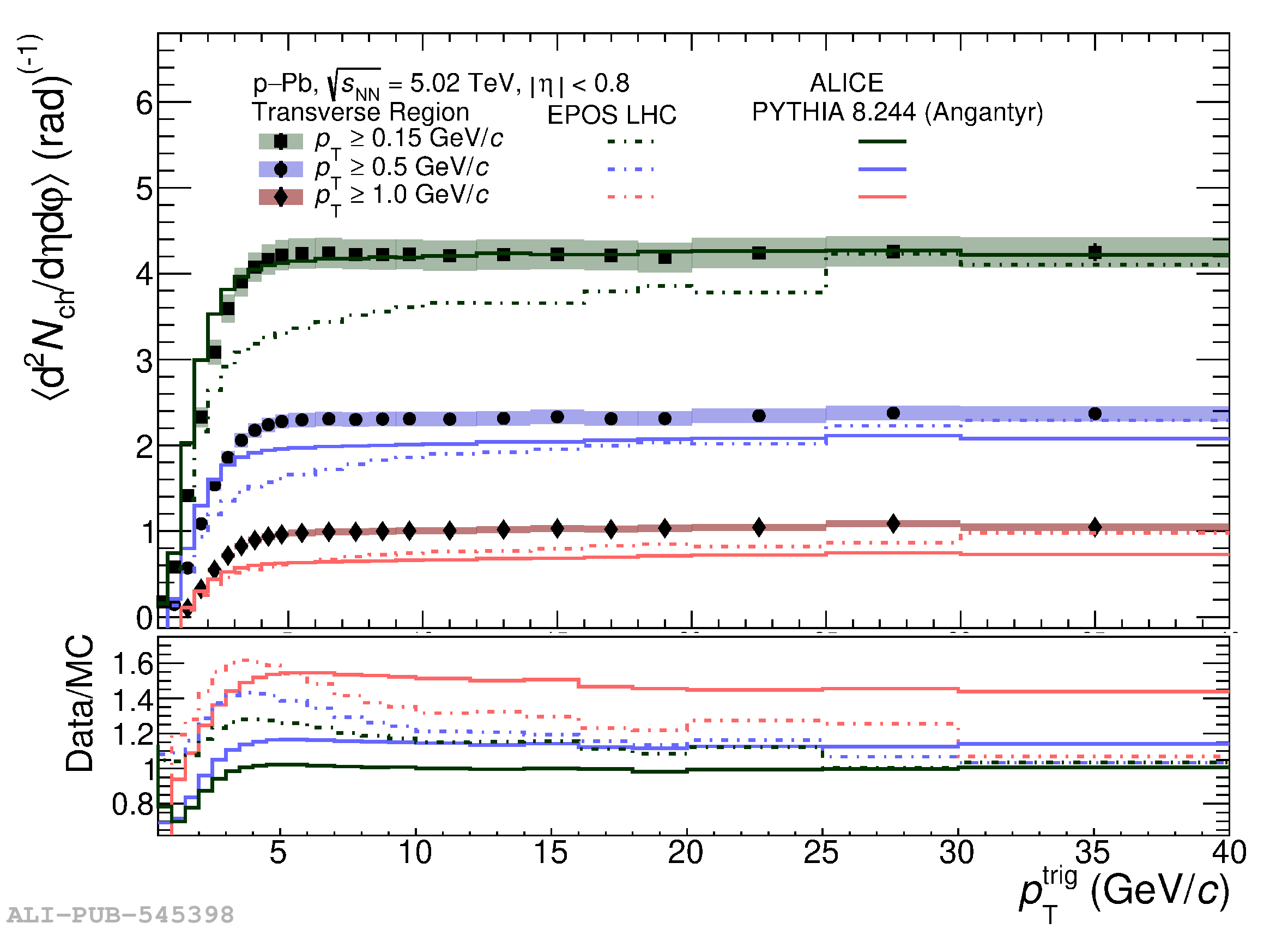# Figure 2

 Charged-particle number density as a function of \ptt measured in the transverse region for \pp (left) and \pPb collisions (right) at $\sqrt{s_{\rm NN}}=5.02$\,\TeV. Measurements were performed considering three \pt thresholds for associated charged particles: $\pt >0.15$\,\GeVc, $\pt >0.5$\,\GeVc, and $\pt >1$\,\GeVc. Data are compared with \py and \ep predictions. The coloured boxes and the error bars represent the systematic and statistical uncertainties, respectively.